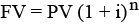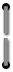# Appendix G

##### HP-80 Calculation Formulas
 Unless otherwise stated: n=Number of time periods i = periodic interest rate expressed as a decimal PMT = payment or deposit PV = present value or principal FV = future value I = interest amount

### Compound Interest##### Annuity### Add on Interest Rate to Annual Percentage Rater = decimal Add on rateAPR = 1200iWhere i is the solution for the above equation.

### Bond Calculations

 n=total number of days between purchase and maturity INTR = decimal coupon rate (on annual basis) YTM = annual yield to maturity in decimal Bond = bond price
##### If n < 182.575